# Maximally-Flat Impedance Transformers

New for September 2021: here's an example of an equal-ripple multi-section transformer using a spreadsheet you can download from our site.

Here we will review the math behind the maximally-flat impedance transformation. The maximally-flat transformer is a multi-section stepped-impedance transformer, where the sections are all one quarter-wavelength at center frequency. This may take some time to post, so be patient!

There's a really great spreadsheet in our download area that performs the maximally-flat impedance transformation for up to eight sections.

### References:

You can learn more about the math by picking up a copy of Matthai Young and Jones, or Pozar, or Rizzi, or Collin's books. Look for them on our book page.

Some of the early work on this topic was authored by Collin, Cohn, Riblett and others in the IRE digests in the 1950s, and during the RadLab days. The foundation for this analysis is called the Theory of Small Reflections which we'll cover later.

## Max-flat transformer: exact solution

The N-section max-flat transformer is maximally flat because the first N derivatives of the frequency response have been set to zero at the center frequency.

For the max flat solution or the binomial approximation, the bandwidth of what you are trying to achieve is not a parameter you get to optimize, it is a function of the number of sections and the ratio of the two impedances you are trying to match. Mostly, you get what you have room for, or how much loss you can stand. That is why many transformers end up being Chebychev, where you get to specify maximum ripple across the band of interest.

This problem is not easy to solve. Early researchers used mainframe computers, solved for the coefficients numerically, and published tables on the topic which are still used today. The Microwaves101 transformer calculator (located in our download area) uses interpolation from an ancient table to provide you with the true max flat solution without the messy math!

More to come!

## Binomial transformer: approximate solution

The binomial transformer is an easy-to-calculate solution to the max-flat transformer problem. In the binomial transformer, the reflection coefficients (and therefore the impedance steps) are related to the binomial coefficient, which you may be familiar with from probability theory, or the binomial transformation of (X+Y)^N.

The binomial transformer provides the exact solution for N=1(which is trivial) and N=2 (which is useful), but higher-order transformers it only offers an approximation. The approximation falls apart rapidly (compared to the exact solution) as mismatch between source and load becomes greater than 2:1, and should not be used. Even though this is just an approximation, and therefore should not be used unless you are in a hurry or don't care about degraded performance, the math is quite elegant. If you disagree with that last statement, go get yourself another snack and then watch some youtube.

### Binomial coefficients

In probability theory, the binomial coefficients are the answer to the question, how many ways can I choose n things from a group of N things? For example, how many ways can you choose 3 objects from 5? The answer is 10. Rather than counting the choices in your head, here's the formula: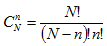Binomial coefficients can also be found using Pascal's triangle, instead of applying the above equation. Blaise Pascal was a 17th century French mathematician, who accomplished much in his short 39 years, but eventually ended up weirded out in a religious cult. The triangle is constructed so each number is equal to the sum of the two numbers directly above it. The edge numbers are all "1" because there are only a single number above them. Below we have plotted the Pascal's triangle up to the 10th row. We didn't use a calculator, contact us if you find any mistakes! Oops, the 200's in the last row should be 210... thanks, Dan! We'll fix that later when we have nothing to do.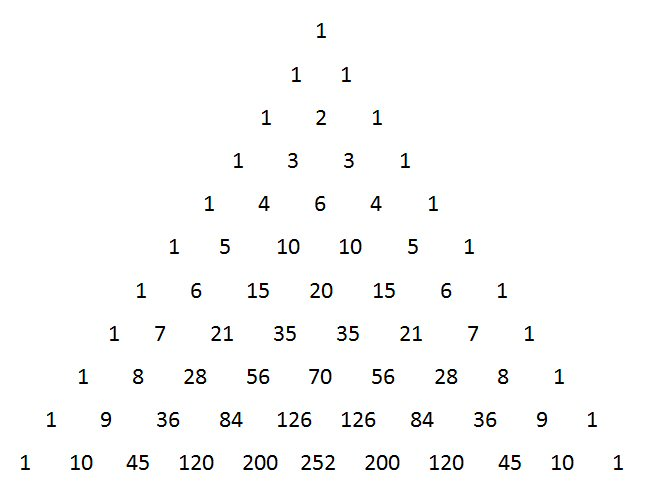Note that C(N,0)=C(N,N)=1 (there's only one way to choose all or nothing). Also the binomial coefficients are symmetric C(N,n)=C(N,N-n), and the coefficients are highest in the middle (the most choices you can have in a deck of 52 cards is when you pick 26).

Below we show how the coefficients are calculated, and how they are related to the binomial expansion. We found the math in Peter Rizzi's book, Microwave Engineering.

The section impedances for the approximate max-flat transformer solution are found from: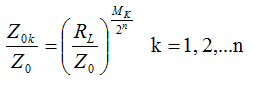Where Mk is related to coefficients Ck which are almost exactly the binomial coefficients. Sorry, we don't have a derivation on how this solution satisfies the max flat transformer, perhaps someday we will. Here's how to calculate Ck: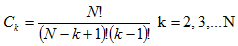Thus Ck is the the array of "N choose n" coefficients, but slightly modified. Below we show the Ck array on Pascal's triangle, the "trick" is that the coefficients start on the second row and omit the last value. Again, the 200's in the last row should read 210 in the graphic...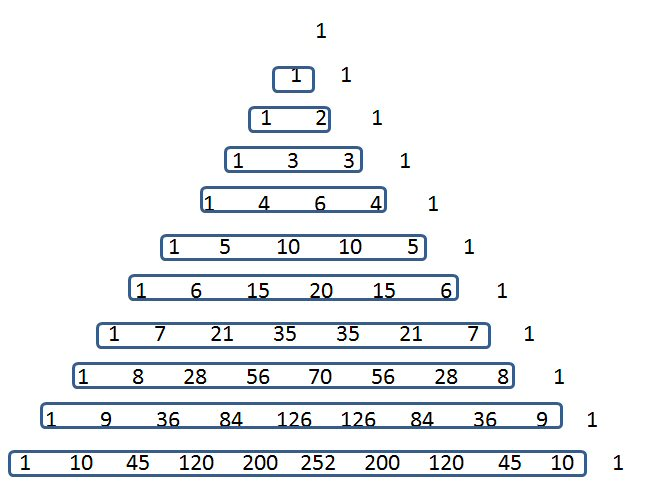An interesting thing about Ck coefficients is that when you sum them for a group of N, the sum is equal to N^2. We'll let you ponder that mystery while we move on to something more useful.

Here is how to calculate the Mk coefficients from the Ck coefficients, this is just a simple summing operation: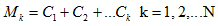Below we have calculated the Ck's and Mk's for binomial transformers up to N=5:

 N Ck Mk 1 C1=1 M1=1 2 C1=1, C2=2 M1=1, M2=3 3 C1=1, C2=3, C3=3 M1=1, M2=4, M3=7 4 C1=1, C2=4, C3=6, C4=4 M1=1, M2=5, M3=11, M4=15 5 C1=1, C2=5, C3=10, C4=10, C4=5 M1=1, M2=6, M3=16, M4=26, M5=31

An interesting thing about binomial coefficients Mk is that when you sum them for a group of N, the sum is equal to N^2. We'll let you ponder that mystery while we move on to something more useful.

Finally, below we have solved for each impedance in terms of the source impedance Z0 and load impedance RL, for up to N=5. Any higher than that will be easy for you to solve, now that we have revealed the pattern!

N=2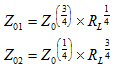N=3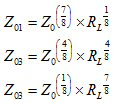N=4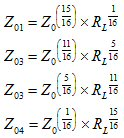N=5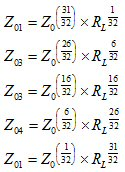### Example 1: N=2, 50 to 100 ohms

Below is the frequency response of an N=2 maximally-flat transformer which was used to match a 2:1 load (100 ohms to 50 ohms). The two section impedances work out to 84.09 ohms and 59.46 ohms. We plotted the response using the Professor's transformer spreadsheet which you can get for free in our download area. By the way, it offers the exact solution to the max-flat problem for up to ten sections (N=10), by interpolating a lookup table which contains data discovered by ancient microwave scholars in the middle of the 20th century!

Why did we pick N=2 for our first example? This is the one case where the approximate formula gives the exact max-flat results. Just don't ask us to prove that statement!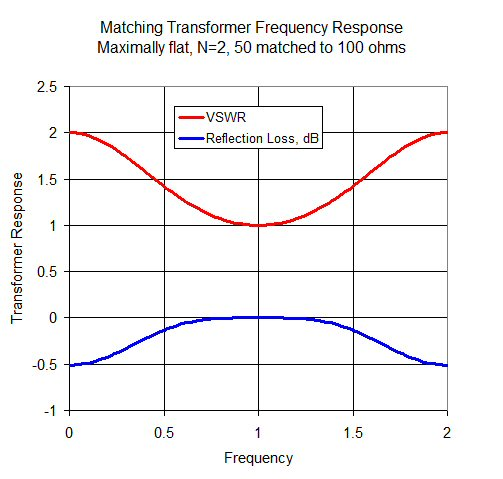So, how close is the binomial approximation to the actual max-flat case? We'll try to answer that in the next two examples...

### Example 2: N=5, 50 to 40 ohm mismatch

Here, we use a five section transformer to match a 40 ohm load to a 50 ohm source. We compare the binomial transformer (port 1) to the max-flat case (port 2). We calculated the max-flat impedance steps using the transformer calculator from our download area. The binomial equations are easy to enter into a VAR block in an ADS simulation, as shown.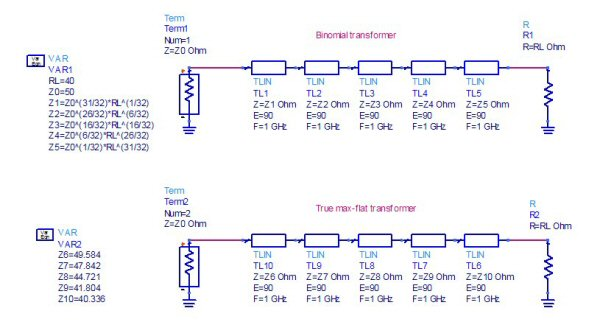Here's the line impedances compared. Z1 is close to the 50 ohms generator. Notice they are almost the same values, so close it would be impossible to fabricate transformers with impedances controlled enough to actually witness the differences. The center impedance (Z3) is the exact same value for both, in both types of transformers for odd values of N, the center section impedance is the geometric mean between the mismatched impedances.

 Z1 Z2 Z3 Z4 Z5 Binomial 49.653 47.951 44.721 41.709 40.280 Max flat 49.584 47.842 44.721 41.804 40.336

Here's the reflection coefficients compared. The two transformers are almost identical, but the max-flat (blue line) has just a small advantage in bandwidth.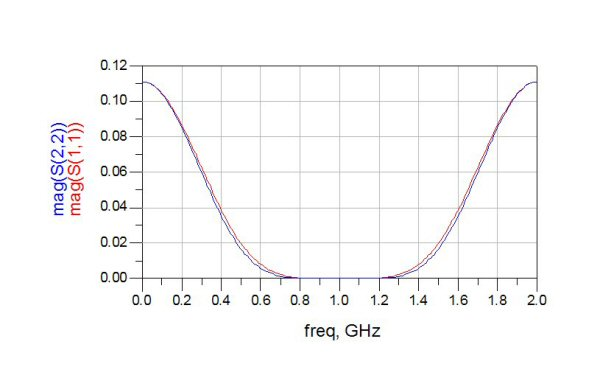Below are the VSWRs of the two transformers. At"DC" it is easy to see that the mismatch is 1.25:1 for both (50:40 ohms).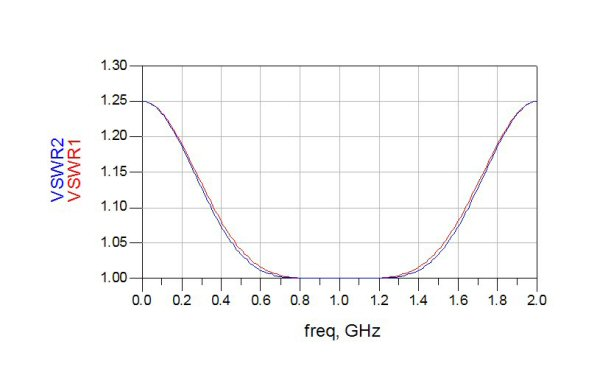### Example 3: N=5, 50 to 40 ohm mismatch

Here we've increased the mismatch to 5:1 (50 to 10 ohm transformation). Again, the binomial transformer is represented by port 1, while max flat is port 2. The max-flat impedances came from our downloadable transformer Excel file.

 Z1 Z2 Z3 Z4 Z5 Binomial 47.547 36.975 22.361 13.522 10.516 Max flat 47.294 36.612 22.361 13.657 10.572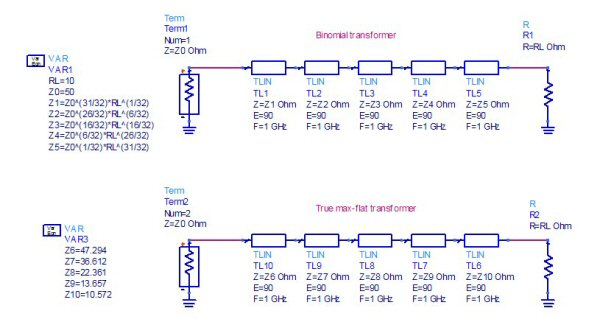Again, the reflection coefficient is zero at center frequency, and the binomial transformer behaves very nearly like the max-flat.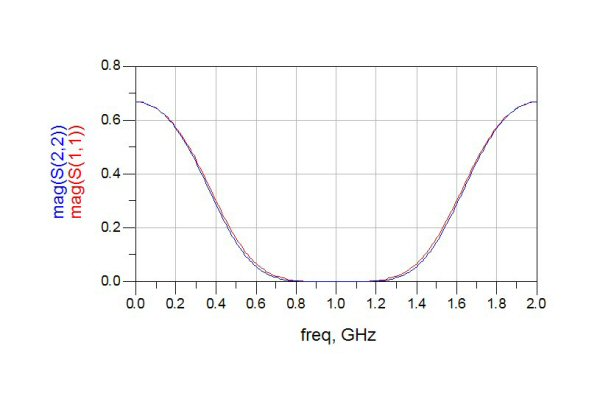Here's the comparison in terms of VSWR; at DC the 5:1 mismatch makes sense (50:10 ohms).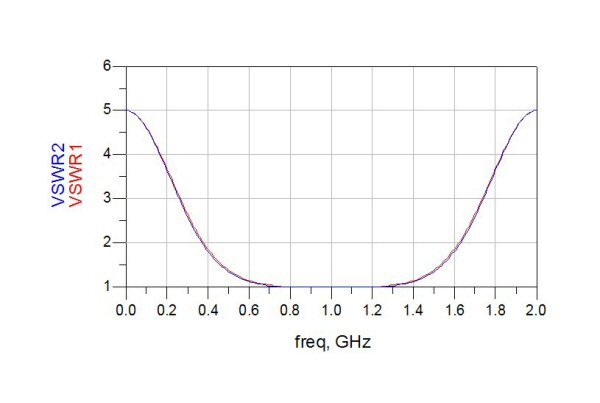In conclusion, since we don't see an appreciable difference, we recommend using the binomial transformer as it is so convenient to calculate. On the other hand, maybe something is incorrect with the max-flat calculation in the downloadable spreadsheet... we'll have to check this out and get back to you. If anyone has any comments send them our way!

Author : Unknown Editor